Faster R-CNN 提出 Region Proposal Network(RPN)，与检测网络共享整张图片的卷积特征，region proposal 计算量几乎很少.

RPN 是全卷积网络，能够同时预测 object 边界和每个位置 object 的 score.

RPNs 是 end-to-end 训练的，以生成高质量的 region proposals.

Fast R-CNN 利用 region proposals 来进行目标检测.

## 1. 回顾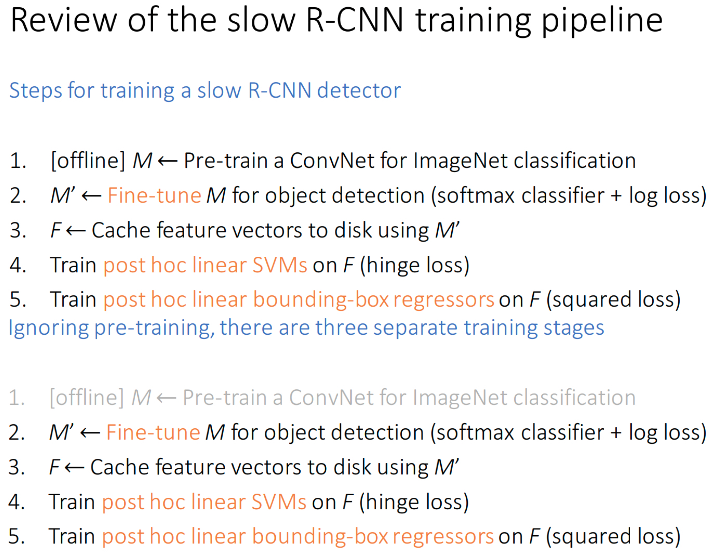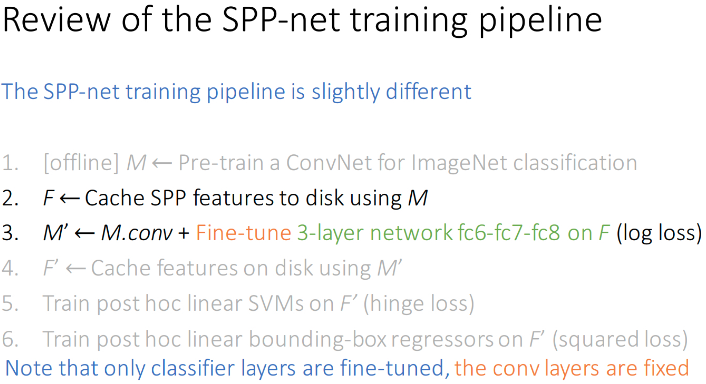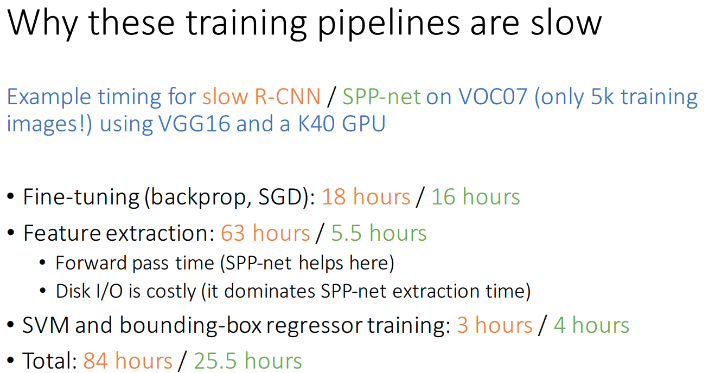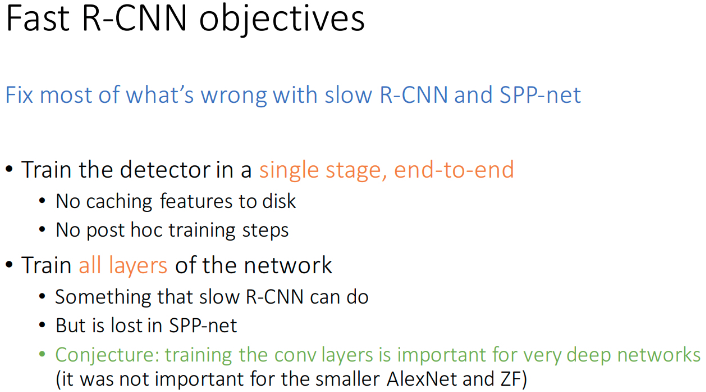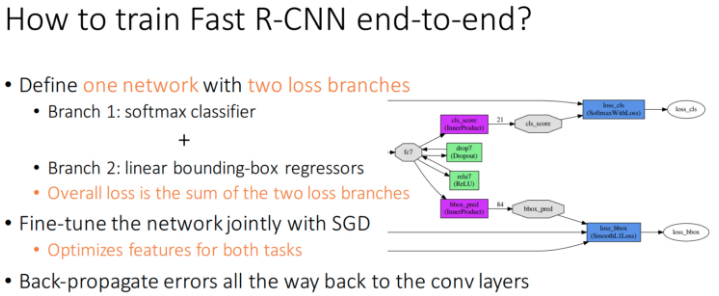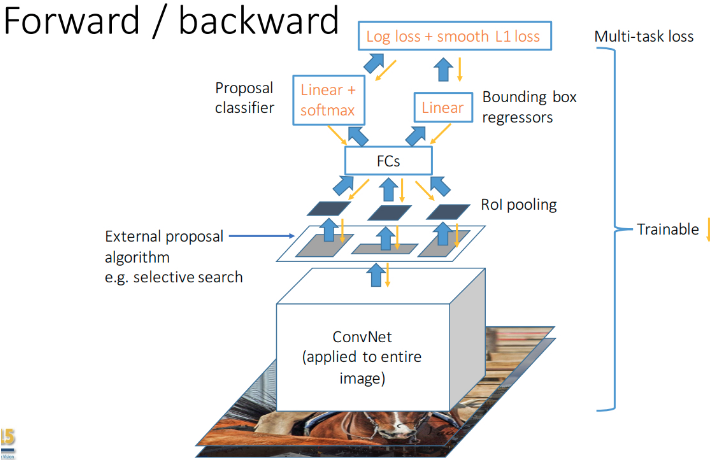End-to-end 训练的好处:

• 实现简单;
• 训练速度更快

• 不需要再将特征写入磁盘, 并再从磁盘读取; 减少 IO .
• 不需要 SVMs 和 bounding-box 回归器单独训练.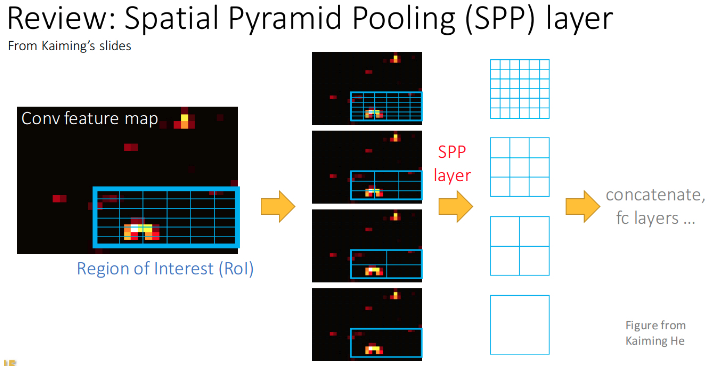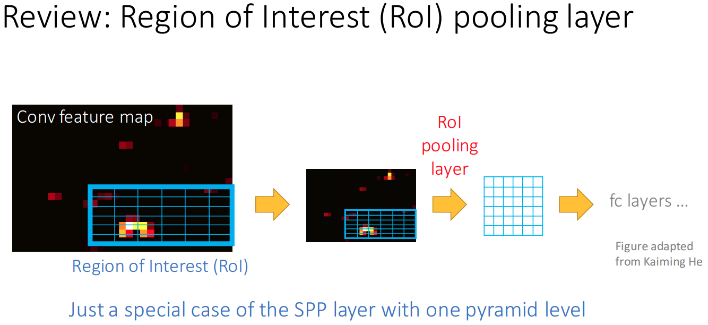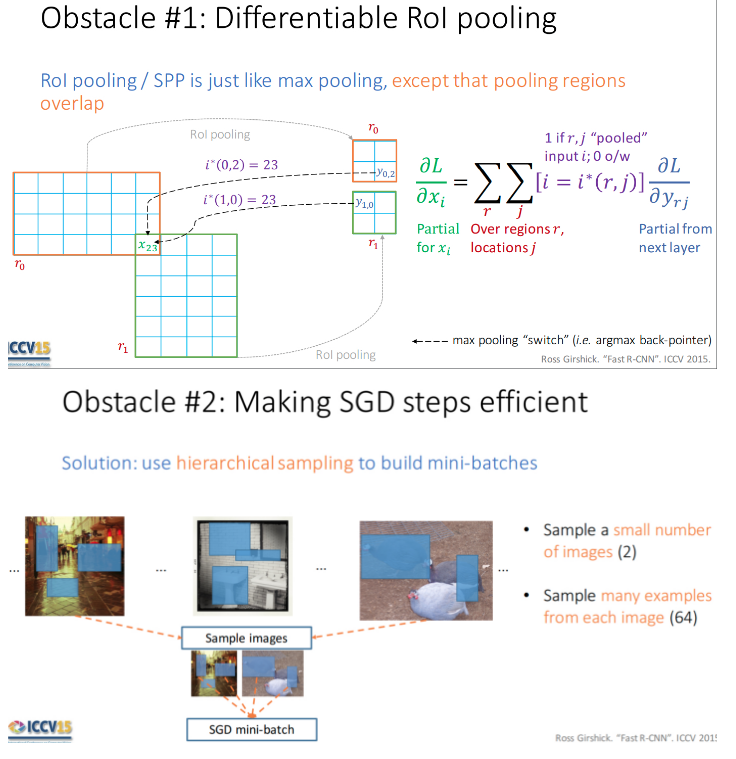## 2. Faster R-CNN

Faster R-CNN 的基本结构• Conv Layers - 输入是 image，输出是提取的图片的 feature maps，被用作 RPN 和全连接层的共享特征；
• RPN - 基于 feature maps 来生成 region proposals. 主要是对 anchors 采用 softmax 来确定其是 foreground 或 background，并对 anchors 进行 bounding box 回归，进而获得理想的 proposals；
• RoI Pooling - 输入是 feature map 和 proposals，输出是提取的 proposal feature maps，被用于全连接层对类别判定；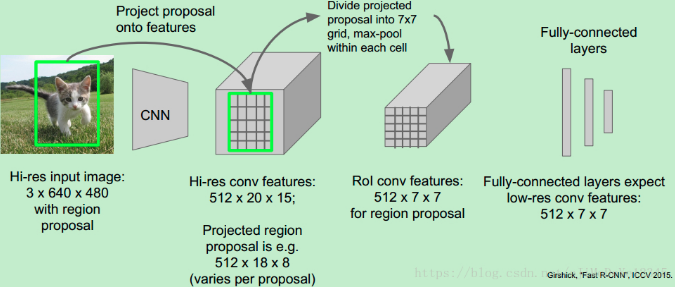• Classifier - 基于 proposal feature maps 来对 proposal 的类别进行计算，并再次进行 bounding box 回归，以得到准确的 object 检测框位置.

## 3. Region Proposal Networks(RPN)

region-based 检测器(如 Fast R-CNN)用到的卷积特征图(feature maps)，也可以用来生成 region proposals.

RPN 采用任意尺寸的图片作为输入，并输出 object proposals 的矩形框集合，每个矩形框都有一个 object score.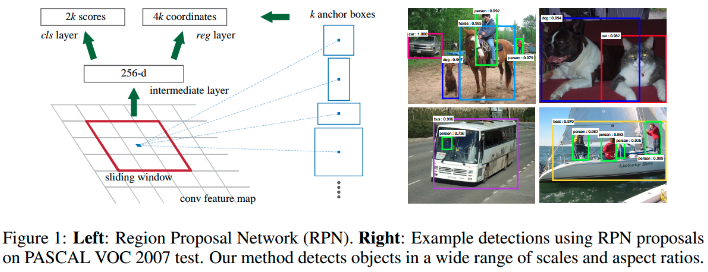• 针对最后一个共享卷积层输出的 conv feature map，采用一个小网络对其平滑.该小网络全连接到输入 conv feature map 的一个 n×n 的空间窗口(spatial window). 这里 n=3.n×n 的卷积层后接 ReLUs 层.
• 每个滑窗被映射为一个低维向量(256-d/ZF，512-d/VGG).
• 低维向量被输入到两个并列 1×1 的卷积层 --边界框回归层(_reg_) 和边界框分类层(_cls_).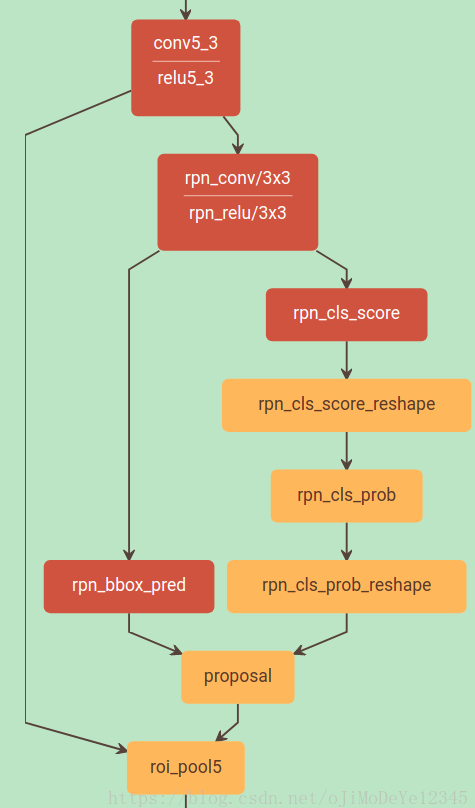### 3.1. 平移不变 Anchors

k 个 proposals 相对于 k 个参考 boxes 进行参数化，记为 anchors，其是一组矩形框. 每个 anchor 在滑窗的中心，并与一个 scale 和 aspect ratio 相关. 这里采用 3 个 scales 和 3 个 aspect ratios，在每个滑窗位置得到 k=9 个anchors.

Faster R-CNN 的一个重要属性是，对于 anchors 和计算相对于 anchors 的 proposals 的函数，都具有平移不变性.

Faster R-CNN 参数更少，在小数据集上过拟合的风险更低.

### 3.2. Region Proposals 学习的 Loss 函数

• 与 groundtruth box 间的 IoU 最大的 anchor(s)；
• 与 groundtruth box 间的 IoU 大于 0.7 的 anchor.

positive 和 negative 的 anchor 不影响训练目标函数.• i - mini-batch 内 anchor 的索引；
• ${ p_i }$ - anchor i 是某个 object 的预测概率；
• ${ p_i^{\ast} }$ - 如果 anchor 是 positive，则 groundtruth label ${ p_i^{\ast} = 1 }$；如果 anchor 是 negative，则 groundtruth label ${ p_i^{\ast} = 0 }$；
• ${ t_i }$ - 表示预测边界框的 4 个参数化坐标的向量；
• ${ t_i^{\ast} }$ - 对应于 positive anchor 的 groundtruth 边界框的 4 个坐标的向量；
• ${ L_{cls}(p_i, p_i^{\ast}) }$ - 二类 softmax loss
• ${ L_{reg}(t_i, t_i^{\ast}) = R(t_i- t_i^{\ast}) }$ - ${ R }$ 是 smooth L1 loss.
• ${ p_i^{\ast}L_{reg} }$ - 表示只有 positive anchor(${ p_i^{\ast} = 1}$) 时，回归loss 才会激活；如果${ p_i^{\ast} = 0 }$，则其值为 0.

cls 层和 reg 层的输出分别包含 ${ \lbrace p_i \rbrace }$ 和 ${ \lbrace t_i \rbrace }$，这两项通过 ${ N_{cls} }$ 和 ${ N_{reg} }$ 进行归一化，并加入平衡权重 ${ \lambda }$.x,y,w,h 分别表示 box 中心的两个坐标，box 的 width 和 height.
${ x, x_a, x^{\ast} }$ 分别为预测 box，anchor box 和 groundtruth box.

### 3.3. 优化

• RPN，全卷积网络，可以利用 SGD 和 BP 进行 end-to-end 的训练；
• image-centric 的采样策略；
• 每个 mini-batch 由包含许多 positive 和 negative anchors 的单张图片构成；
• 随机采样一张图片的 256 个 anchors 来计算 mini-batch 的 loss，采样的 positive anchors 和 negative anchors 的比例为 1:1；如果一张图片的 positive anchors 少于 128，则用 negative anchors 来补全 mini-batch.
• 采用均值为 0，方差为0.01 的 Gaussian 分布来初始化网络新加入的层；
• 采用 ImageNet 分类任务的预训练模型来初始化其它网络层；
• PASCAL 数据集上，前 60K 次 mini-batches，learning_rate=0.001，后 20K 次迭代，learning_rate=0.0001；
• momentum=0.9，weight_decay=0.0005.

### 3.4. Region Proposal 和目标检测共享卷积层特征

4-step 训练算法，以通过交替优化来学习共享特征：

 - 训练 RPN；采用 ImageNet 训练模型进行网络初始化，并针对 region proposal 任务进行 end-to-end 的 fine-tuned.

 - 基于 RPN 生成的 proposals，采用 Fast R-CNN 来训练一个单独的检测网络；检测网络也是采用 ImageNet 训练模型初始化；此时，两个网络是不共享卷积层的.

 - 采用检测网络来初始化 RPN 的训练，但固定共享卷积层，只 fine-tune RPN 的网络层；现在，两个网络共享卷积层.

 - 固定共享卷积层，fine-tune Fast R-CNN 的 FC 层.

### 3.5. 实现细节

• 采用 single-scale 图片来训练和测试 region proposal 和 object detection 网络；
• 将图片短边 rescale 到 600 像素；
• 针对 anchors，采用 3 种 scale —— box 面积分别为 $128^2$，$256^2$，$512^2$ 像素, 3 种 aspect ratios -- 1:1，1:2，1:3.
• 跨图像边界的 anchor boxes 的处理.
训练时，忽略所有的 cross-boundary anchors，因此，其不会影响 loss.

• 由于 RPN proposals 会高度重叠，这里采用 NMS 基于 proposal regions 的 cls scores 进行处理；
固定 NMS 的 IoU 阈值为 0.7，每张图片大约能保留 2k proposal regions.
• NMS 处理后，采用 top-N proposal regions 进行目标检测.

## 4. 训练网络

models/coco/VGG16/faster_rcnn_end2end/train.prototxt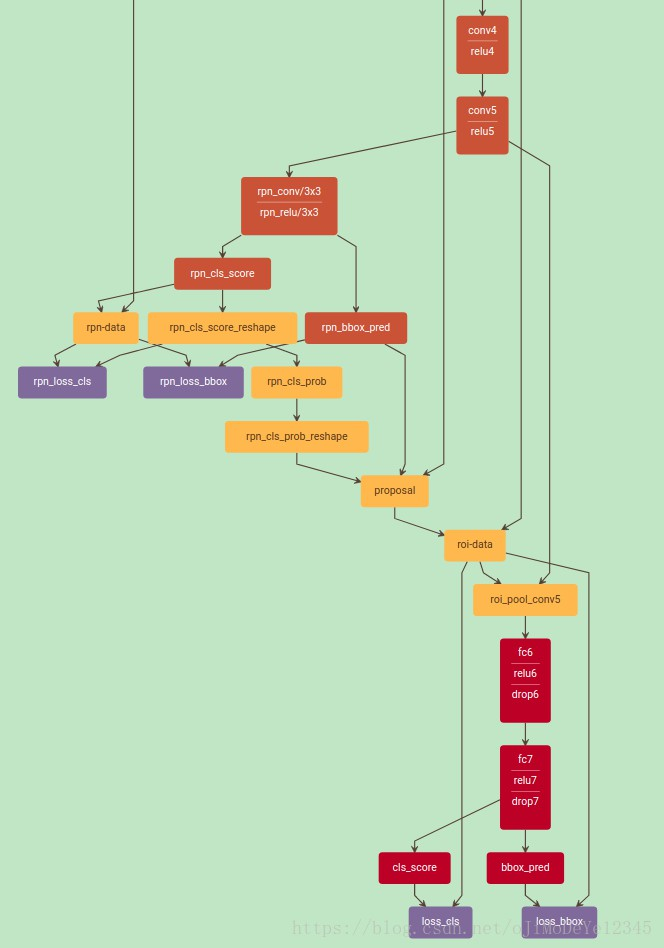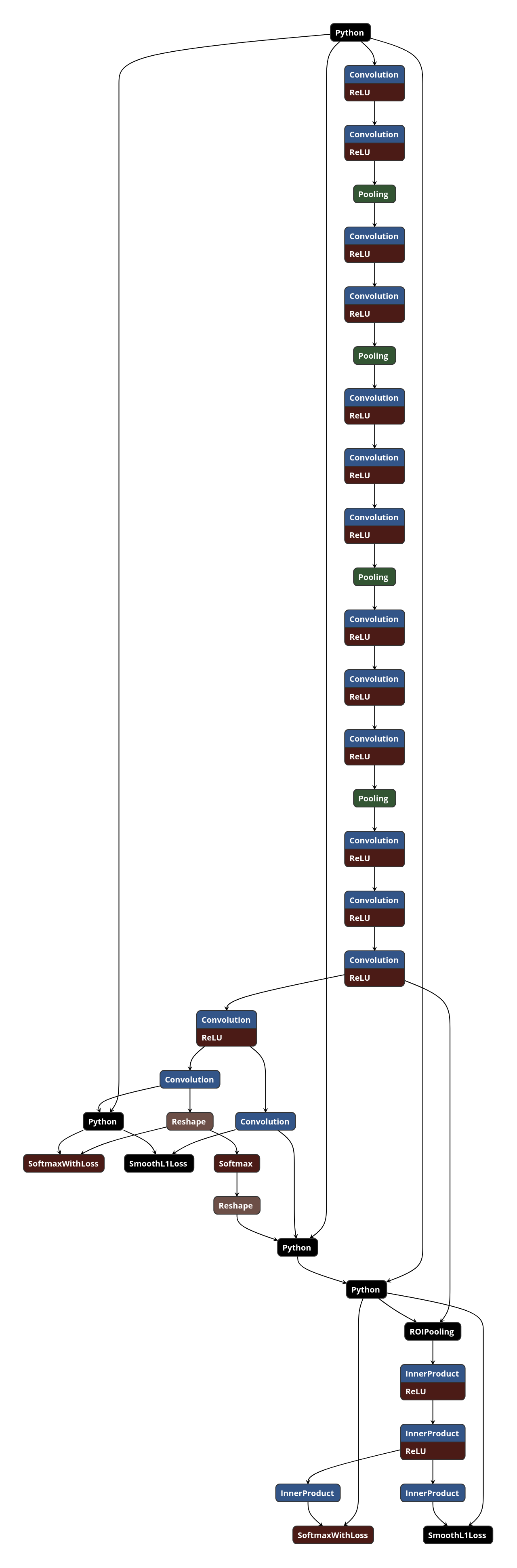## 5. 测试网络

models/coco/VGG16/faster_rcnn_end2end/test.prototxt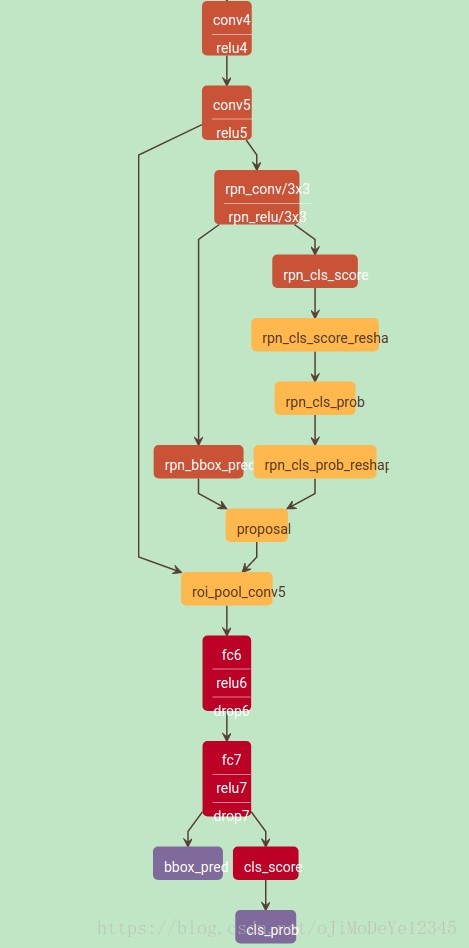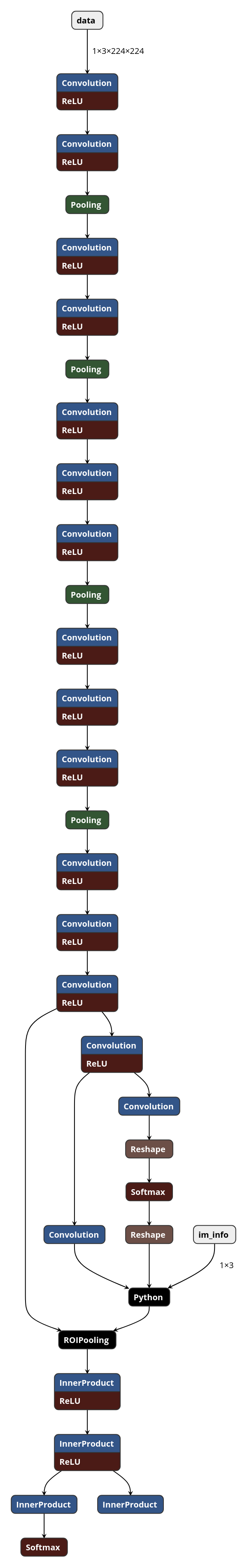## 6. Reference

Last modification：May 10th, 2019 at 01:04 pm